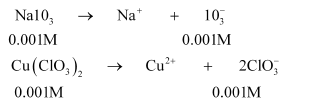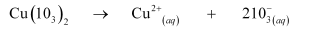# Equal volumes of 0.002 M solutions of sodium iodate and cupric chlorate are mixed together.`
Question:

Equal volumes of $0.002 \mathrm{M}$ solutions of sodium iodate and cupric chlorate are mixed together. Will it lead to precipitation of copper iodate? (For cupric iodate $K_{\text {sp }}=7.4 \times 10^{-8}$ ).

Solution:

When equal volumes of sodium iodate and cupric chlorate solutions are mixed together, then the molar concentrations of both solutions are reduced to half i.e., 0.001 M.

Then,Now, the solubility equilibrium for copper iodate can be written as:Ionic product of copper iodate

$=\left[\mathrm{Cu}^{2+}\right]\left[10_{3}^{-}\right]^{2}$

$=(0.001)(0.001)^{2}$

$=1 \times 10^{-9}$

Since the ionic product $\left(1 \times 10^{-9}\right)$ is less than $K_{s p}\left(7.4 \times 10^{-8}\right)$, precipitation will not occur.